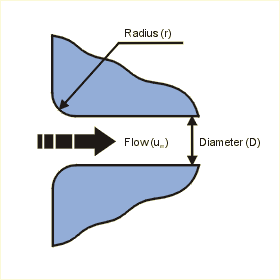Close

The head loss hL can be calculated as:

hL = kloss x (um2 / (2 x g) )

Where kloss is the head loss coefficient, um is the mean flow velocity in the pipe, and g is the gravitational acceleration. kloss is calculated from:

kloss = 235.03x4 - 194.09x3 + 56.739x2 - 7.8163x + 0.50

Where x is the defined as:

x = r / Dh

Where r is the radius of the pipe and Dh is the hydraulic diameter.The kloss equation was derived from a curve fit from points when plotted from the following data and are accurate plus/minus 10%:

 r/Dh kloss 0.00 0.5 0.01 0.44 0.02 0.37 0.03 0.31 0.04 0.26 0.05 0.22 0.06 0.2 0.08 0.15 0.10 0.12 0.12 0.09 0.16 0.06 0.20 0.03

References

Roberson, John A. and Crowe, Clayton T., Engineering Fluid Mechanics, 2nd Ed., Rudolf Steiner Press, London.1976. p 360 - 384.

Idel'chik, I E. and Fried, Erwin., Flow Resistance, a Design Guide for Engineers, Taylor & Francis, Washington D.C. 1989. p 52.

ASHRAE. ASHRAE Handbook, 1977 Fundamentals, American Society of Heating, Refrigerating And Air Conditioning Engineers, Inc., New York, 1997. p 32.31.